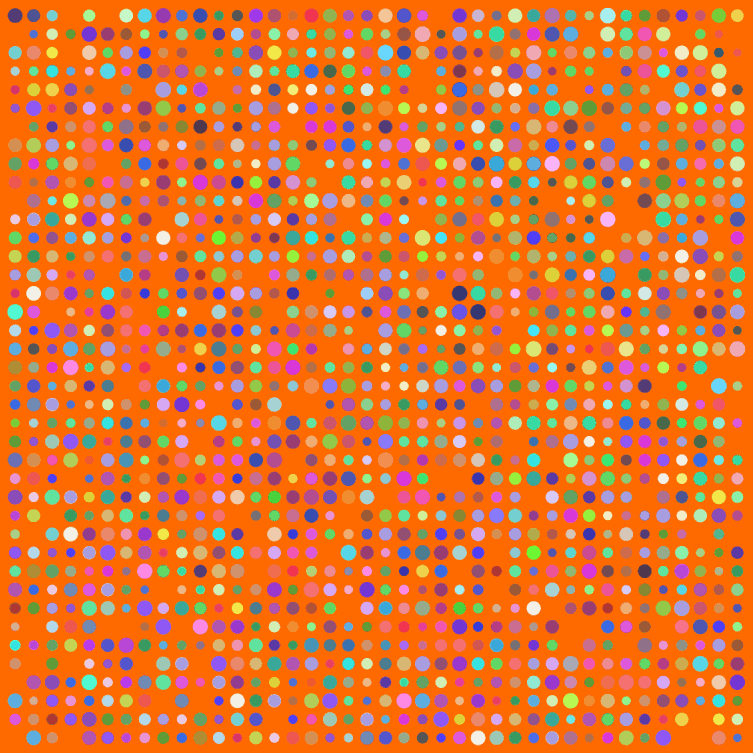[This article was first published on Probability and statistics blog » r, and kindly contributed to R-bloggers]. (You can report issue about the content on this page here)
Want to share your content on R-bloggers? click here if you have a blog, or here if you don't.I have a few posts nearing completion, but meanwhile a weekend break for art. Big thanks to Simon Urbanek and Jeffrey Horner, creators of Cairo, a library for the programming language R. Have you noticed how R can’t anti-alias (fancy way for saying smooth out lines and curves when creating a bit-mapped image)? Cairo can.

Make sure to click the image above for the full version. Here’s my code:

```# The Cairo library produces nice, smooth graphics Cairo(1200, 1200, file="D:/Your/Path/Here/Dots.png", type="png", bg="#FF6A00")   # How big should the grid for placing dots be? myWidth=40 myHeight=40   dotsPlaced = myWidth*myHeight   # Optional default colors and sizes for dots myColors = rep(c("#0000F0","#00F000"),dotsPlaced) myCex = rep(3.2,dotsPlaced)   for(i in 1:dotsPlaced) { # Change this to allow more of the default color dots to survive if(runif(1)<1) { myColors[i] = paste("#",paste(sample(c(3:9,"A","B","C","D","E","F"),6,replace=T),collapse=""),collapse="",sep="") } myCex[i] = runif(1,3,6) }   # Keeping this is marginal par(oma=c(0,0,0,0)) par(mar=c(0,0,0,0))   # Start off with a blank plot. The white dot helps with cropping later plot(0,0,pch=".",xlim=c(0,40),ylim=c(0,40),col="white", xaxt = "n", yaxt = "n")   for(m in 1:myWidth) { for(n in 1:myHeight) { if(runif(1) < .93) { points(n,m,pch=20,col=myColors[((m*n)+n)],cex=myCex[((m*n)+n)]) } } }   dev.off() # Tell Cairo to burn the plot to disk```